# C++ Program to Display Armstrong Number Between Two Numbers

In this tutorial you will learn about the C++ Program to Display Armstrong Number Between Two Numbers and its application with practical example.

## C++ Program to Display Armstrong Number Between Two Numbers

In this tutorial, we will learn to create a C++ program that will Display Armstrong Number Between Two Numbers in C++ programming.

## Prerequisites.

Before starting with this tutorial we assume that you are best aware of the following C++ programming topics:

• Operators in C++ Programming.
• Basic Input and Output function in C++ Programming.
• Basic C++ programming.
• While loop in C++ programming.

## Program to Display Armstrong Number Between Two Numbers:-

In today’s tutorial, we will create a program that will find the Armstrong numbers between the given number range. First will take the number frame from the user and then will find the Armstrong Number.

With the help of this program, we can Display Armstrong Number Between Two Numbers.

## Output:-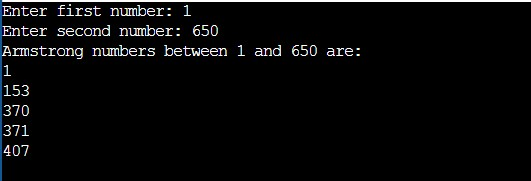In the above program, we have first initialized the required variable.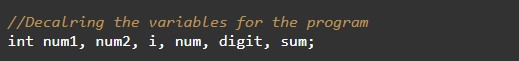• num1 = it will hold the integer value.
• num2 = it will hold the integer value.
• flag = it will hold the integer value.
• digit = it will hold the integer value.
• num = it will hold the integer value.
• sum = it will hold the integer value.
• i = it will hold the integer value.

Taking the input integer number interval from the user.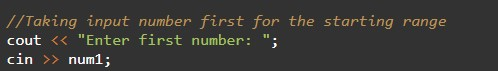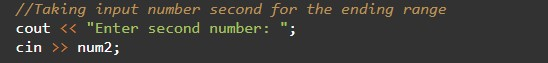Calculating the Armstrong numbers.Printing the output numbers.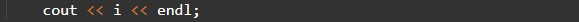In this tutorial we have learn about the C++ Program to Display Armstrong Number Between Two Numbers and its application with practical example. I hope you will like this tutorial.